# How to Write Net Ionic Equations in Chemistry?

How to Write Net Ionic EquationsHave you ever watched a cricket match in a stadium? Of course, you must have. Where there are bowlers, batsmen, and the umpire, actually participating in the match, there are also tonnes of spectators just like you, watching the match, cheering for their favorite team but not actually participating in the sport.

Well, there is a very close relevance of this example in chemistry, especially when writing net ionic equations. Whenever a chemical reaction takes place and a complete ionic equation is written, this complete ion equation represents all the ions present while the reaction takes place.

A simplified version of this complete ionic equation is a net ionic equation that represents only the ions actually taking part in the chemical reaction, eliminating spectator ions.

In this article, we will talk about everything you need to know about net ionic equations with lots of examples of how to write these net ionic equations in chemistry.

But before that, let us go through some basic definitions related to these concepts.

 ❖  What are ionic compounds?Ionic compounds are compounds that break down into their constituent ions in an aqueous solution. ❖  What is a complete ionic equation?A complete ionic equation is used to represent the ionic compounds present in a chemical reaction. All the ionic compounds participating in a chemical reaction are shown as dissociated ions in a complete ionic equation.There are two types of ions present in the complete ionic equation i.e., the ions actually participating in the reaction called the participating ions or principal species versus the spectator ions. ❖  What are spectator ions?Spectator ions are positively charged (cations) or negatively charged (anions) ions that do not participate in the chemical reaction. Rather, they stay unchanged on both the reactant and the product side of the complete ionic equation.❖  What is a net ionic equation?A net ionic equation is a simplified version of the complete ionic equation. The spectator ions are canceled out on both the reactant and the product side. In this way, the net ionic equation shows only those elements, compounds or ions that are directly involved in the chemical reaction.Different state symbols are used to represent the physical state of compounds in the complete and net ionic equations such as (s) for solid, (l) for liquid, (aq) for aqueous, and (g) for gaseous reactants and/or products. ❖  What are precipitates and aqueous solutions?The precipitates are defined as the solid products obtained in a chemical reaction. The aqueous solution is a solution prepared in water. A solution is made up of a solute and a solvent. If water is used as the solvent, then that solution is known as an aqueous solution.While writing an ionic equation, the compounds present in an aqueous solution will dissociate into their respective ions while the solid precipitates will stay un-dissociated.
Page Contents

## Difference between a complete ionic equation and a net ionic equation ?

 Complete ionic equation Net ionic equation Represents all the dissociated ions Represents only the ions actually involved in a chemical reaction The spectator ions are present The spectator ions are canceled out from the reactant and the product sides

Thus, the net ionic equation is important because it represents only the active ions participating in the chemical reaction.

Net ionic equations are particularly important for representing acid-base neutralization reactions, redox reactions, double displacement reactions etc.

Let’s understand how to write a net ionic equation with the help of some examples.

## How to write a net ionic equation?

You can follow the following simple steps while writing a net ionic equation.

1. Write the complete ionic equation from the chemical reaction equation.
2. Identify the spectator ions from the complete ionic equation.
3. Cancel out the spectator ions on both sides of the equation.
4. Obtain the net ionic equation.Let’s understand the process of writing net ionic equations with the help of examples.

### Step 1: Write the complete ionic equation from the chemical reaction equation.

For example, a chemical reaction takes place between copper (II) sulfate and sodium chloride in aqueous forms.

The chemical formula for copper (II) sulfate is CuSO4 while the chemical formula for sodium chloride is NaCl.

The chemical reaction between CuSO4 and NaCl can be represented by the balanced chemical equation shown below.On the reactant side, CuSO4 dissociates into Cu2+ and SO42- ions in an aqueous solution. Similarly, 2 NaCl dissociates into 2 Na+ and 2 Cl ions in the aqueous solution.

On the product side, Na2SO4 dissociates into 2 Na+ and SO42- while CuCl2 is a precipitate (it is in the solid form) so it will not dissociate into its respective ions and stay as it is.

So, the chemical equation given above can be written as a complete ionic equation, as shown below.### Step 2: Identify the spectator ions from the complete ionic equation.

In the equation above, you can see that 2 Na+ ions and an SO42- ion stays unchanged on both the reactant and the product sides.

As these ions stay uninvolved during the chemical reaction therefore they are marked as spectator ions.### Step 3: Cancel out the spectator ions on both sides of the equation

Make sure to check that the net ionic equation is a balanced equation.### Step 4: Obtain the net ionic equation

So, the balanced net ionic equation for this reaction is:Using all the steps given above, practice with us some more examples for a better understanding of writing net ionic equations.

## Examples of writing net ionic equations

Example 1

 A complete neutralization reaction takes place between sodium hydroxide (NaOH) and hydrochloric acid (HCl). A balanced chemical equation for the reaction is given below.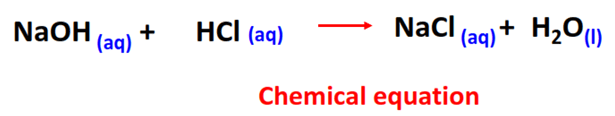NaOH dissociates into Na+ and OH ions while HCl dissociates into H+ and Cl on the reactant side. While on the product side, NaCl is also water soluble so it breaks down into Na+ and Cl ions in the presence of H2O.  So the complete ionic equation for this reaction is:This net ionic equation shows that an acid-base neutralization reaction actually takes place between hydrogen (H+) and hydroxide (OH) ions only.

Example 2

 A neutralization reaction takes place between magnesium hydroxide Mg(OH)2 and sulfuric acid (H2SO4). A balanced chemical equation for the reaction is given below.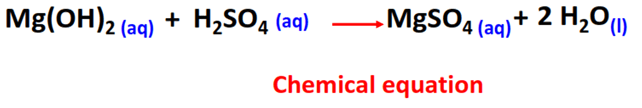Mg(OH)2 dissociates into Mg2+ and 2 OH ions while H2SO4 dissociates into 2 H+ and SO42- on the reactant side. While on the product side, MgSO4 formed is a water-soluble ionic compound so it dissociates into Mg2+ and SO42- ions under aqueous conditions. Thus, the complete ionic equation for this reaction is:Example 3

 Lead (II) nitrate Pb(NO3)2 reacts with potassium iodide (KI) to yield potassium nitrate and a bright yellow precipitate of lead iodide (PbI2).Pb(NO3)2 dissociates into Pb2+ and 2 NO3 ions while 2 KI dissociates into 2 K+ and 2 I ions on the reactant side. 2 KNO3 dissociates into 2 K+ and 2 NO3 ions while PbI2 stays intact on the product side as it is a precipitate. So the complete ionic equation for this reaction is:Example 4

 Sodium chloride (NaCl) reacts with silver nitrate (AgNO3) to yield a white silver chloride (AgCl) precipitate while sodium nitrate (NaNO3) is formed as the by-product.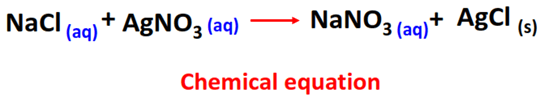NaCl dissociates into Na+ and Cl ions while AgNO3 dissociates into Ag+ and NO3ions on the reactant side. NaNO3 dissociates into Na+ and NO3 ions while AgCl stays intact on the product side. So the complete ionic equation for this reaction is:Notice that we have placed the Ag+ ion first and then the Clion is written in the net ionic equation. This is because the convention is that the positively charged cation is written prior to the negatively charged ion on the reactant side while writing net ionic equations. However, this rule is not compulsory.

You should also keep in mind that in addition to the same moles of an element on both reactant and product sides, the positive and negative charges should also be balanced, as you can ensure from any of the net ionic equations we have discussed in this article.

Now, let’s see another example.

Example 5

 Copper (II) chloride (CuCl2) reacts with sodium phosphate (Na3PO4) to produce a blue solid precipitate known as copper (II) phosphate and sodium chloride.3 CuCl2 dissociates into 3 Cu2+ and 6 Cl ions while 2 Na3PO4 dissociates into 6 Na+ and 2 PO43- ions on the reactant side. 6 NaCl dissociates into 6 Na+ and 6 Cl ions while Cu3(PO4)2 stays unchanged on the product side. So the complete ionic equation for this reaction is:Positive and negative charges on both sides of the ionic equation are balanced.

You may note that the positive and negative charges are also balanced in this net ionic equation i.e., +6 and -6 respectively on both sides of the equation.

Example 6

 Lead nitrate Pb(NO3)2 reacts with lithium bromide (LiBr). As a result, lithium nitrate (LiNO3) and lead bromide (PbBr2) are produced, as shown in the chemical equation given below.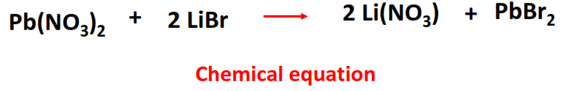Now in the above equation, the physical state symbols of all the reactants and products are not given. In such a situation, you need to identify which of the reactants and products are water soluble while others are not.

Applying the solubility rules, we will identify that lead bromide appears as a white solid as r.t.p. It has very low solubility in water i.e., 0.455 g per 100 g H2O. Therefore, in the above chemical reaction, PbBr2 is the precipitate obtained. All the other compounds are water soluble so they appear as aqueous solutions.

Pb(NO3)2 dissociates into Pb2+ and 2 NO3 ions while 2 LiBr dissociates into 2 Li+ and 2 Br ions on the reactant side. 2 LiNO3 dissociates into 2 Li+ and 2 NO3 ions while PbBr2 stays undissociated on the product side. So the complete ionic equation for this reaction is:Example 7

 A chemical reaction between sodium iodide (NaI) and calcium nitrate Ca(NO3)2 produces an aqueous solution of sodium nitrate (NaNO3) and calcium iodide (CaI2).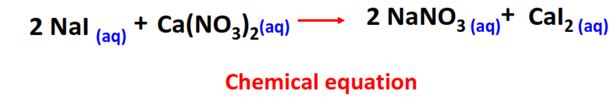Now in this reaction, no precipitates are formed. So, all the ionic compounds will dissociate into their ions both on the reactant as well as the product side.

2 NaI dissociates into 2 Na+ and 2 I ions while Ca(NO3)2 dissociates into Ca2+ and 2 NO3 ions on the reactant side. While on the product side, 2 NaNO3 dissociates into 2 Na+ and 2 NO3 ions while CaI2 breaks down to produce Ca2+ and 2 I ions.All the ions here prove to be spectator ions, so all the ions are canceled out. Thus, there is no such reaction. So no net ionic equation.

Now that you have learned everything about ‘How to write net ionic equations in chemistry’, test your knowledge of the equations given below and let us know you well you performed.

Let’s check:

## FAQ

### What does the net ionic equation represent?

A net ionic equation represents only the ions actually taking part in the chemical reaction, eliminating spectator ions.

### What are the steps involved in writing a net ionic equation?

There are 4 main steps followed for writing a net ionic equation –

• Write the complete ionic equation from the chemical reaction equation.
• Identify the spectator ions from the complete ionic equation.
• Cancel out the spectator ions on both sides of the equation.
• Obtain the net ionic equation.

### What is the difference between a complete ionic equation and a net ionic equation?

A complete ionic equation represents all the dissociated ions whereas the net ionic equation represents only the ions actually involved in a chemical reaction.

The spectator ions are present in the complete ionic equation whereas the spectator ions are canceled out from the reactant and the product sides in the net ionic equation.

### Pb + 2HCl → PbCl2 + H2### Sn + H2SO4 → SnSO4 + H2## Summary

•  The net ionic equation shows only those elements, compounds, or ions that are directly involved in the chemical reaction.
• To write the net ionic equation – follow the steps – (1).  Write the complete ionic equation (2). Identify the spectator ions (3). Cancel out the spectator ions (4). Obtain the net ionic equation.
• The net ionic equation represents only the ions actually taking part in the chemical reaction, eliminating spectator ions.
• A complete ionic equation represents all the dissociated ions.

## Practice Questions on the Net ionic equationDid you like it?

#### Ammara Waheed

Ammara Waheed is a highly qualified and experienced chemist, whose passion for Chemistry is evident in her writing. With a Bachelor of Science (Hons.) and Master of Philosophy (M. Phil) in Physical and Analytical Chemistry from Government College University (GCU) Lahore, Pakistan, with a hands-on laboratory experience in the Pakistan Council of Scientific and Industrial Research (PCSIR), Ammara has a solid educational foundation in her field. She comes from a distinguished research background and she documents her research endeavors for reputable journals such as Wiley and Elsevier. Her deep knowledge and expertise in the field of Chemistry make her a trusted and reliable authority in her profession. Let's connect - https://www.researchgate.net/profile/Ammara-Waheed

Share it...

Topblogtenz is a website dedicated to providing informative and engaging content related to the field of chemistry and science. We aim to make complex subjects, like chemistry, approachable and enjoyable for everyone.